Home > CCA2 > Chapter 12 > Lesson 12.1.1 > Problem12-12

12-12.
1. If you remember what n! means, you can do some messy calculations quickly or compute problems that are too large for your calculator’s memory. For instance, if you wanted to calculate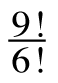, you could use the n! button on your calculator and find that 9! = 362,880 and 6! = 720, so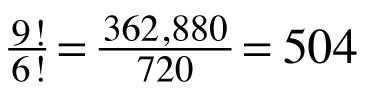. You could also use a simplification technique. Since 9! = 9 ⋅ 8 ⋅ 7 ⋅ 6 ⋅ 5 ⋅ 4 ⋅ 3 ⋅ 2 ⋅ 1 and 6! = 6 ⋅ 5 ⋅ 4 ⋅ 3 ⋅ 2 ⋅ 1, you can rewrite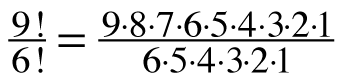= 9 ⋅ 8 ⋅ 7 = 504.

2. Use this simplification technique to simplify each of the following problems before computing the result. Homework Help ✎

1.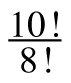2.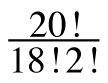3.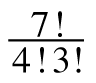4.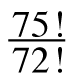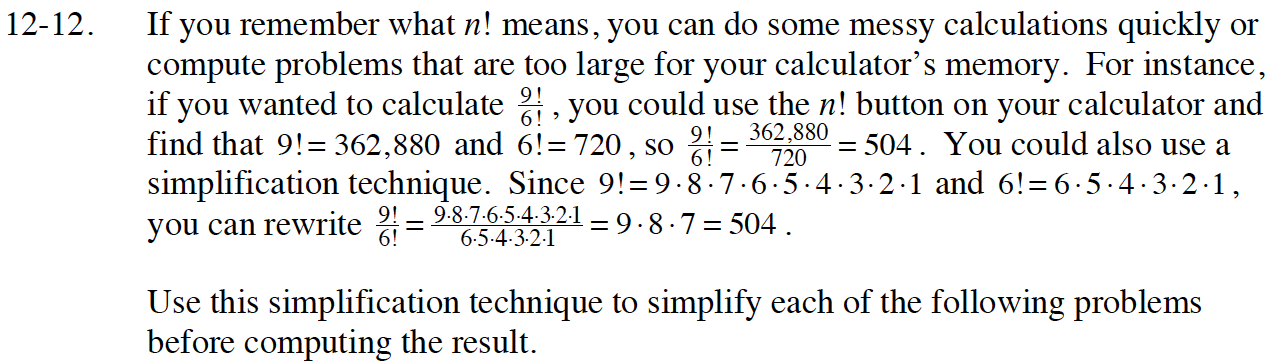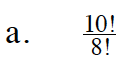90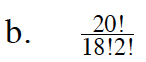$\frac{20\cdot19}{2\cdot1}$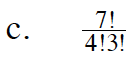Refer to part (b). Remember to write factors for both 4! and 3!.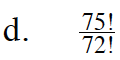In this case, it would be very tedious to write out all the factors.
Think of all the Giant Ones you would create if you did write it all out. What is left?